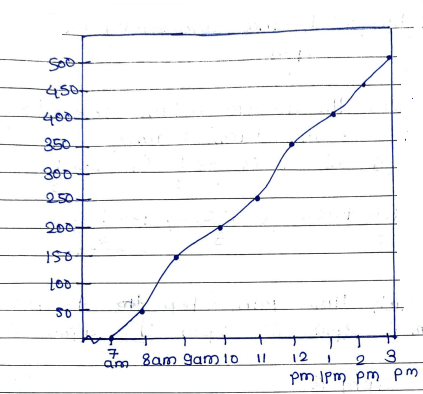# NCERT Book Introducing to graphs MCQ Questions Class 8 Maths Chapter 15

## Introducing to graphs MCQ Questions Class 8 Maths Chapter 15

#### Introducing to graphs

Question 1:The given graph describes the distance covered by Ram at different times. He starts driving at 7 am and give the answers o following questions.

What was the distance covered by ram at 2pm

(a) 500

(b) 450

(c) 600

(d) 250

Ans:

Distance covered by ram at 2pm is 450 km (b) 450.

Question 2:

Ram covered distance 250 km at which time?

(a) 9 am

(b) 10 am

(c) 1 pm

(d) 11 am

Ans:

At 11am (d), Ram covered distance 250 km.

Question 3:

What was the distance covered by ram at 3 pm?

(a) 300

(b) 400

(c) 500

(d) 200

Ans:

500 km (c) Distance covered by ram at 3 pm

Question 4:

What was the average distance covered by ram at 8 am, 9am, 10am?

(a) 200/3

(b) 33

(c) 144 1/3

(d) 133 1/3

Ans:

50+150+200/3 = 400/3 = 133 1/3 (d)

Question 5:

At which time Ram stop driving?

(a) 12 PM

(b) 2 PM

(c) 3 PM

(d) 4 PM

Ans:

At 3 PM (c).

Question 6:Given graph represents the temperature of city and give the answers of following question.

What was recorded temperature of delhi?

(a) 15°

(b) 20°

(c) 25°

(d) 30°

Ans:

15°, recorded temperature of Delhi is 15° (a)

Question 7:

What was recorded temperature of manila?

(a) 10°

(b) 6°

(c) 5°

(d) 15°

Ans:

5° (c) temperature of manila 5°

Question 8:

Highest temperature recorded in which city?

(a) Delhi

(b) Jammu

(c) Chandrapur

(d) Manila

Ans:

Chandrapur.

Question 9:

Lowest temperature recorded in which city?

(a) Jammu

(b) Manila

(c) Delhi

(d) both a & b

Ans:

Both Jammu and manila (d)

Question 10:

Find the average temperature of city Bombay, Jammu and Manila?

(a) 35°

(b) 20°

(c) 15°

(d) 10°

Ans:

35°+5°+5°/3 = 45°/3 = 15° (c)

Question 11:

Plot the following points on a graph sheet A (1, 4) B (1, 3), C (3, 2), d (4, 2)Ans:

(d) In option (d) plot the graph perfectly.

Question 12:

Plot the following points on a graph sheet P (4, 3), Q (1, 3) R (3, 2) S (2, 1)Ans:

The graph in option (c) plot perfectly.

Question 13:

Graph obtained by joining the planted points is a line. Such graph are called

(a) Independent variable

(b) Linear graphs

(c) Curve graph

(d) Origin

Ans:

(b) Linear graphs.

Question 14:

Draw the graph for the following tables of values with suitable scales on the axes.

Cost of mango

 No. of mangoes 1 2 3 4 5 Cost in (₹) 10 20 30 40 50Ans:

In option (d) the graph plot correctly as per given.

Question 15:

Plot a pie chart of tv channels by informative 10%, news, 15% sports 25%, entertainment 50%Ans:

(b) In option (b) pie chart correctly as per given.

Here is your solution of Introducing to graphs MCQ Questions Class 8 Maths Chapter 15

Dear Student, I appreciate your efforts and hard work that you all had put in. Thank you for being concerned with us and I wish you for your continued success.

Updated: April 11, 2023 — 12:44 pm